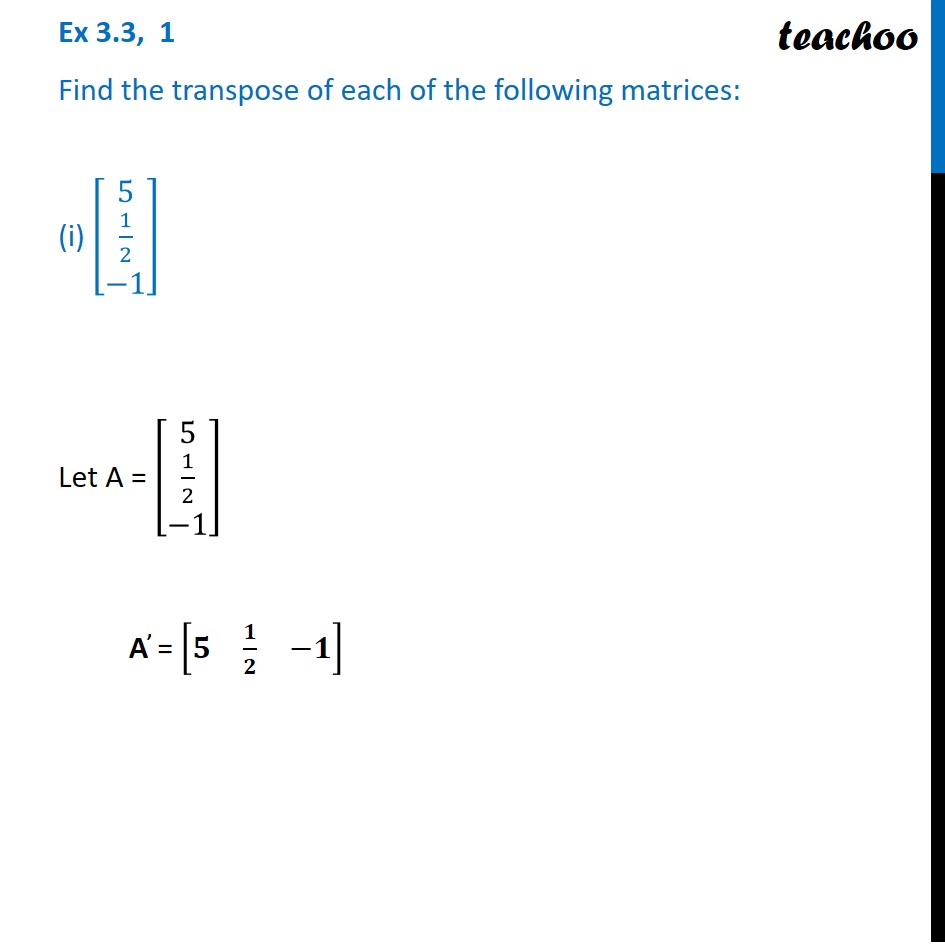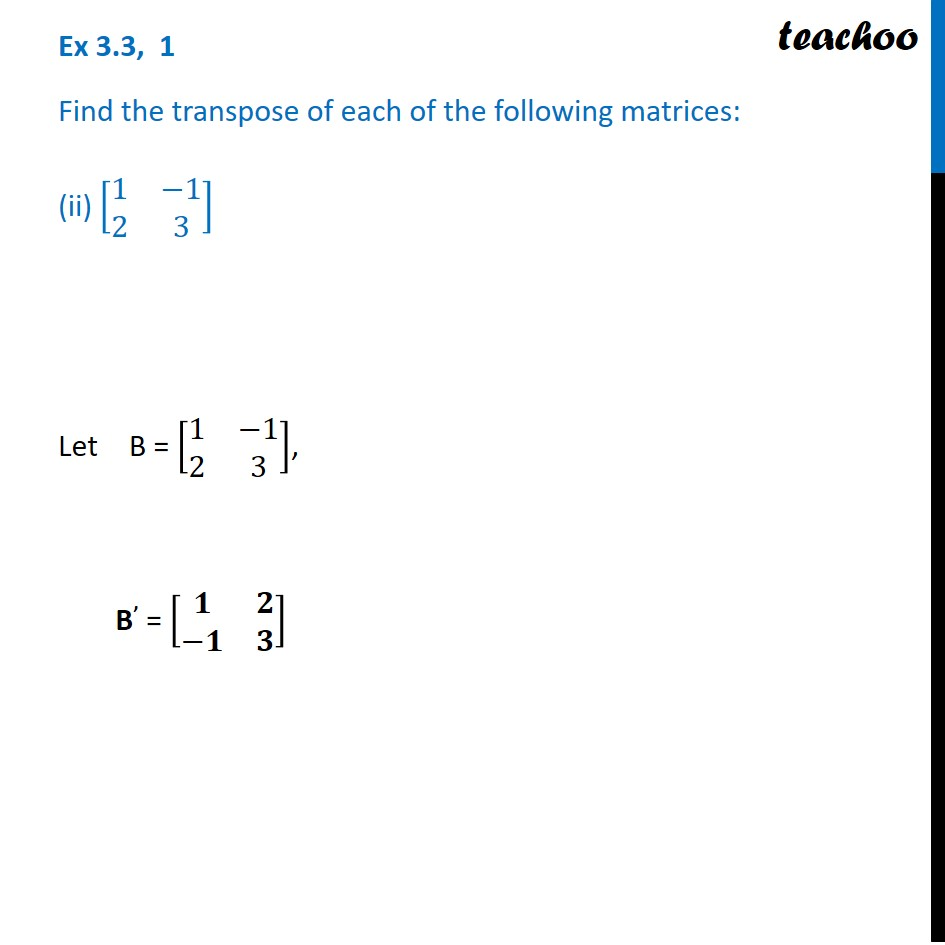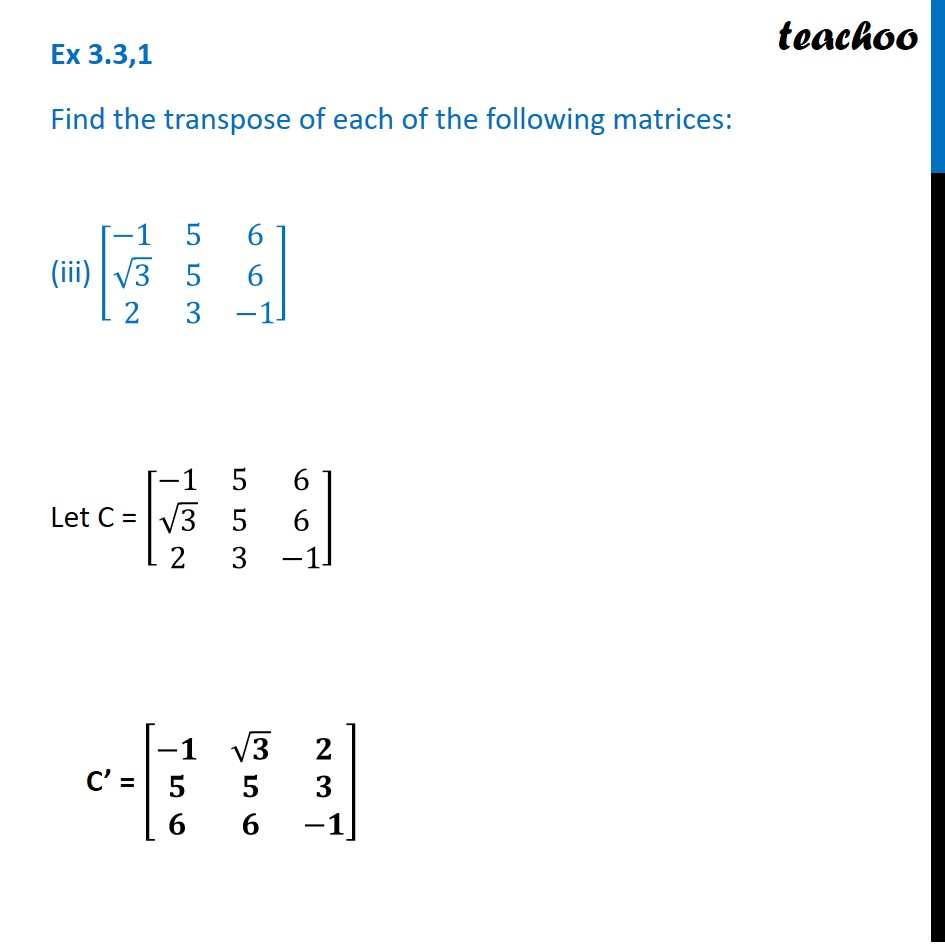Transpose of a matrix

Chapter 3 Class 12 Matrices (Term 1)
Concept wise### Transcript

Ex 3.3, 1 Find the transpose of each of the following matrices: (i) [■8(5@1/2@−1)] Let A = [■8(5@1/2@−1)] A’ = [■8(𝟓&𝟏/𝟐&−𝟏)] Ex 3.3, 1 Find the transpose of each of the following matrices: (ii) [■8(1&−1@2&3)] Let B = [■8(1&−1@2&3)], B’ = [■8(𝟏&𝟐@−𝟏&𝟑)] Ex 3.3,1 Find the transpose of each of the following matrices: (iii) [■8(−1&5&6@√3&5&6@2&3&−1)] Let C = [■8(−1&5&6@√3&5&6@2&3&−1)] C’ = [■8(−𝟏&√𝟑&𝟐@𝟓&𝟓&𝟑@𝟔&𝟔&−𝟏)]Ex 3.3,1 Find the transpose of each of the following matrices: (iii) [■8(−1&5&6@√3&5&6@2&3&−1)] Let C = [■8(−1&5&6@√3&5&6@2&3&−1)] C’ = [■8(−𝟏&√𝟑&𝟐@𝟓&𝟓&𝟑@𝟔&𝟔&−𝟏)]